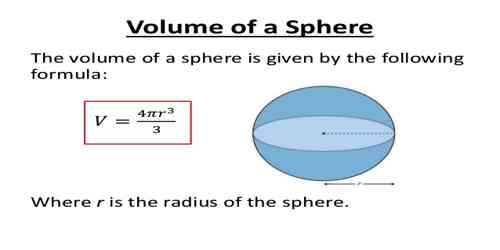Volume of Sphere means the number of cubic units that will exactly fill a sphere.

The volume of sphere can be found by the formula: volume = 4/3 π r3

Where r is the radius of the sphere. In the figure above, drag the orange dot to change the radius of the sphere and note how the formula is used to calculate the volume. Since the 4, 3 and pi are constants, this simplifies to approximately: 4.19 r3

This formula was discovered over two thousand years ago by the Greek philosopher Archimedes. He also realized that the volume of a sphere is exactly two thirds the volume of its circumscribed cylinder, which is the smallest cylinder that can contain the sphere.

The volume of a 3-dimensional solid is the amount of space it occupies. Volume is measured in cubic units (in3, ft3, cm3, m3 etc). Be sure that all of the measurements are in the same unit before computing the volume.

The volume V of a sphere is four-thirds times pi times the radius cubed.

V = 4/3 πr3

The volume of a hemisphere is one-half the volume of the related sphere.

Note: The volume of a sphere is 2/32/3 of the volume of a cylinder with same radius, and height equal to the diameter.Example: Find the volume of the sphere. Round to the nearest cubic meter.

Solution

The formula for the volume of a sphere is- V = 4/3 πr3

From the figure, the radius of the sphere is 8 m.

Substitute 8 for r in the formula.

V = 4/3π(8)3

Simplify.

V = 4/3 π (512) ≈ 2145

Therefore, the volume of the sphere is about 2145 m3.

Information Source: